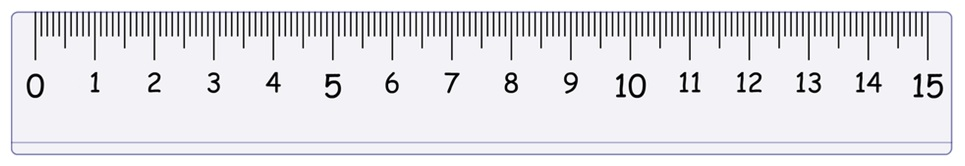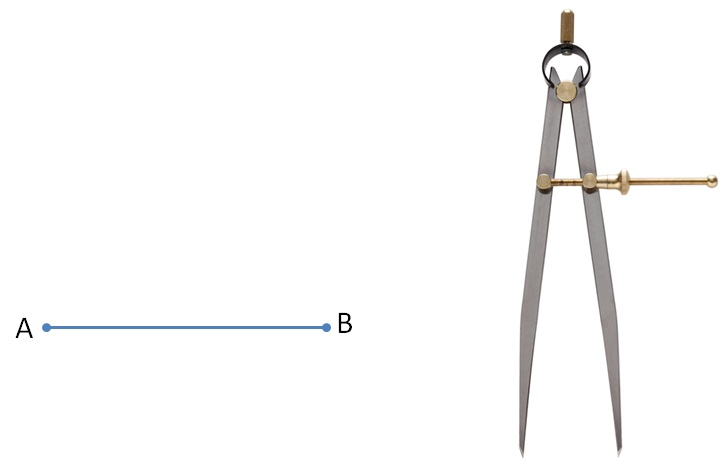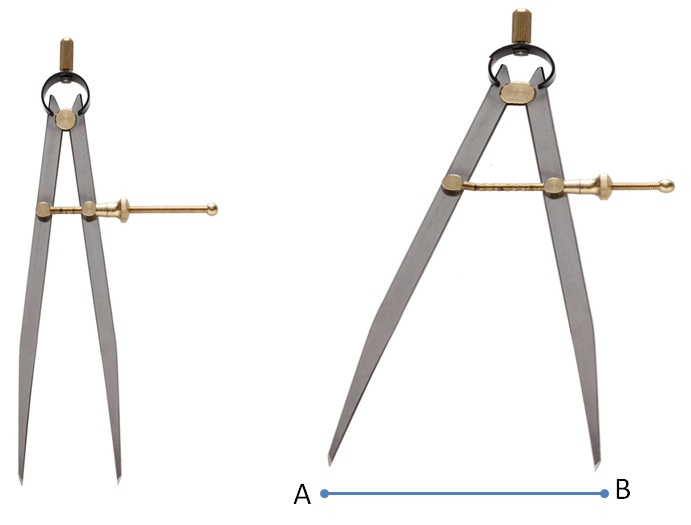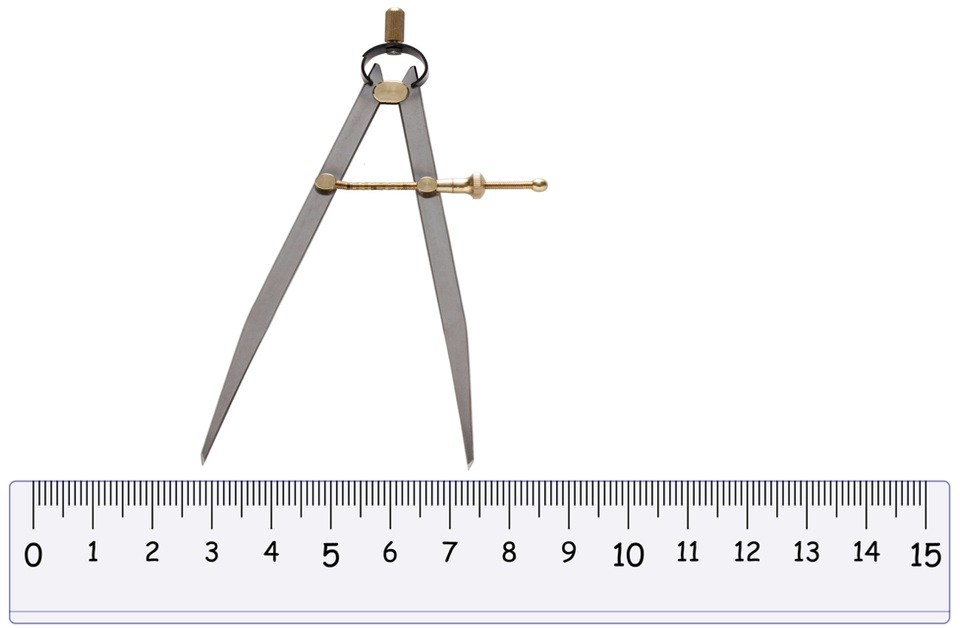Suppose we want to measure line segment ABSteps are

(1) First ,we take a ruler & put its two end points at the end points of the line .(2)   Then use the rule to measure the distance between the two ends of the divider.1. Chapter 5 Class 6 Understanding Elementary Shapes
2. Concept wise
3. Measuring Line Segments

Measuring Line Segments